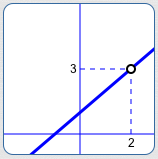﻿ Puncture Points (Holes)

#Puncture Points (Holes)

Sometimes, students are fooled into thinking that a rational function has a vertical asymptote when—in reality—it has a puncture point (also called a ‘hole’).

This section provides detail and practice with punctured graphs.A graph with a ‘puncture point’ (also called a ‘hole’)

First, an example:

## Does the function $\,R(x) = \frac{x^2 - x - 2}{x - 2}\,$ have a vertical asymptote at $\,x = 2\,$? No!

Some well-intentioned web sites conclude that this function has a vertical asymptote at $\,x = 2\,,$ simply because the denominator is zero there. This is incorrect.

In order to find a vertical asymptote, it is NOT ENOUGH to just set the denominator equal to zero.

You must ALSO check that the numerator is nonzero!

For this particular function, substitution of $\,x = 2\,$ into the numerator gives:

$$\cssId{s14}{2^2 - 2 - 2\ = \ 4 - 2 - 2 \ = \ 0}$$

Therefore, both the numerator and denominator are zero when $\,x = 2\,.$

When BOTH the numerator and denominator of a rational function are zero, then you must work harder to figure out what's going on.

You must determine what's happening CLOSE TO the $x$-value of interest.

Further analysis shows:

\begin{align} \cssId{s19}{R(x)}\ &\cssId{s20}{= \frac{x^2-x-2}{x-2}}\cr\cr &\cssId{s21}{= \frac{(x+1)(x-2)}{x-2}}\cr\cr &\cssId{s22}{= (x+1)\cdot\frac{x-2}{x-2}} \end{align}

Cancelling off the extra factor of one:

$$\cssId{s24}{R(x) = x + 1\,,}\ \cssId{s25}{\text{providing } x\ne 2}$$

The function $\,R\,$ isn't defined when $\,x = 2\,,$ since division by zero isn't allowed.

For all values of $\,x\ne 2\,,$ ‘$\,\frac{x-2}{x-2}\,$’ is just the number $\,1\,$! The graph of $\,R\,$ is therefore the same as the line $\,y = x + 1\,,$ except that it has been ‘punctured’ at $\,x = 2\,.$

From this re-naming, it is now clear that when $\,x\,$ is close to $\,2\,,$ the outputs are close to $\,2 + 1 = 3\,.$ The graph of the function $\,R\,$ is shown below:DEFINITION puncture points (holes)

Let $\displaystyle\,R(x) = \frac{N(x)}{D(x)}\,$ be a function.

The function $\,R\,$ has a puncture point (hole) at $\,x = c\,$ if and only if the following three conditions hold:

• $D(c) = 0\,$   (the denominator is zero at $\,c\,$)
• $N(c) = 0\,$   (the numerator is zero at $\,c\,$)
• As $\,x\,$ approaches $\,c\,,$ the values $\,R(x)\,$ approach a finite number

## Notes About Puncture Points (Holes)

### This Definition Holds for Rational Functions, and More!

It might be that both $\,N\,$ and $\,D\,$ are polynomials, making this a rational function (i.e., a ratio of polynomials). However, $\,N\,$ and $\,D\,$ could be more general functions.

For example, a bit of calculus shows that the function $\displaystyle\,R(x) = \frac{{\text{e}}^{x-2} - 1}{x - 2}\,$ has a hole at $\,x = 2\,.$

Note: In calculus, a ‘hole’ is an example of a removable discontinuity.

### You Need All Three Conditions

It it not enough merely to require that both the numerator and denominator are zero.

Why? Instead of a puncture point, you might have a vertical asymptote.

For example, the function $$R(x) = \frac{x-2}{x^2 - 4x + 4} = \frac{x-2}{(x-2)(x-2)}$$ has a vertical asymptote at $\,x = 2\,.$

Why? For all $\,x\ne 2\,,$ $\displaystyle\,R(x) = \frac{1}{x-2}\,.$

### Typical Approach for Analyzing a Rational Function for Vertical Asymptotes/Holes

1. Set the denominator equal to zero.
2. Check the resulting value(s) in the numerator.
3. If the denominator is zero and the numerator is nonzero, then you're guaranteed to have a vertical asymptote.
4. If the numerator and denominator are both zero, then you must do some additional analysis: you have either a vertical asymptote or a hole.